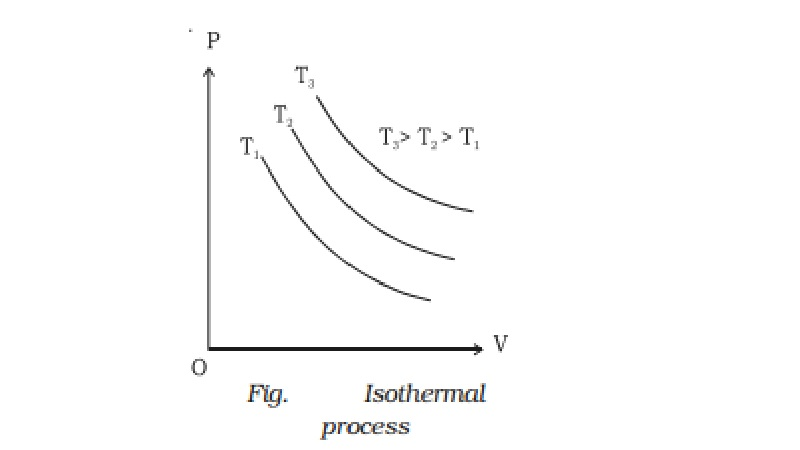Home | | Physics | | Physics | Isothermal process and Workdone in an isothermal expansion

# Isothermal process and Workdone in an isothermal expansionWhen a gas undergoes expansion or compression at constant temperature, the process is called isothermal process.

Isothermal process

When a gas undergoes expansion or compression at constant temperature, the process is called isothermal process.

Let us consider a gas in a cylinder provided with a frictionless piston. The cylinder and the piston are made up of conducting material. If the piston is pushed down slowly, the heat energy produced will be quickly transmitted to the surroundings. Hence, the temperature remains constant but the pressure of the gas increases and its volume decreases.

The equation for an isothermal process is PV = constant.If a graph is drawn between P and V, keeping temperature constant, we get a curve called an isothermal curve. Isotherms for three different temperatures T1, T2 and T3 are shown in the Fig.. The curve moves away from the origin at higher temperatures.

During an isothermal change, the specific heat capacity of the gas is infinite.

( i.e)  C = ∆Q/m ∆T = infinity

(e.g) Melting of ice at its melting point and vapourisation of water at its boiling point.

Workdone in an isothermal expansion

Consider one mole of an ideal gas enclosed in a cylinder with perfectly conducting walls and fitted with a perfectly frictionless and conducting piston. Let P1, V1 and T be the initial pressure, volume and temperature of the gas. Let the gas expand to a volume V2 when pressure reduces to P2, at constant temperature T. At any instant during expansion let the pressure of the gas be P. If A is the area of cross section of the piston, then force F = P ? A.

Let us assume that the pressure of the gas remains constant during an infinitesimally small outward displacement dx of the piston. Work done

dW = Fdx = PAdx = PdV

Total work done by the gas in expansion from initial volume V1 to final volume V2 is

W = ∫v1v2 PdV

We know, PV = RT, P = RT/V

W =  ∫v1v2 RT/V dV =  RT v1v2 1/V dV

W = RT [logeV]v1v2

W = RT [logeV2 - logeV1]

= RTloge (V2/V1)

This is the equation for the workdone during an isothermal process.

Study Material, Lecturing Notes, Assignment, Reference, Wiki description explanation, brief detail

Related Topics